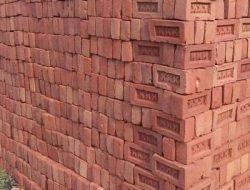### Calculate Number of Bricks for Walls, Columns, Foundations

This brick calculator provides the calculation for number of bricks required for masonry walls, columns, foundations etc. Also, how to calculate number of bricks manually is discussed.

## Standard Sizes of Bricks

Standard sizes of bricks in India are 190mm x 90mm x 90mm. and in United States is 7.5inch x 3.5 inch x 3.5 inch without mortar. In the calculator below, you can input your bricks sizes to find the number of bricks.## How to Calculate Number of Bricks for Masonry Works

A brick masonry construction uses mortar as binding material for bricks. The volume of mortar used in masonry works generally ranges from 25 to 30 % of brick masonry volume.
The thickness of mortar in brickwork is generally assumed to be 10mm or ½ inch thick. With mortar thickness, the size of such a brick becomes 200 mm x 100 mm x 100 mm (8 inch x 4 inch x 4 inch) and it is known as the nominal size of the modular brick.
For calculating the number of bricks, the thickness of plaster should be deducted from the thickness of masonry construction. For example, for a brick wall of 230mm thick, the actual thickness without plaster would be 200mm, i.e. one brick thick.

### Number of Bricks for Masonry Wall

#### In Metric System:

Consider a wall with 5m length, 4m height and 200mm thickness.
Volume of brick masonry in wall = 5 x 4 x 0.2 = 4m3
Volume of one brick with mortar = 0.2 x 0.1 x 0.1 = 0.002 m3
Number of bricks = [Volume of brick masonry / volume of one brick] = 4 / 0.002 = 2000 bricks.
Thus, number of bricks required per cubic meter = 500.

#### In United States Standard Feet System:

Consider a wall with 15 feet length, 10 feet height and 8 inch thickness.
Volume of brick masonry in wall = 15 x 10 x 8/12 = 100 cubic feet = 3.704 cubic yard
Volume of one brick with mortar = 8 x 4 x 4 = 128 cubic inch = 0.074074 cubic feet
Number of bricks = [Volume of brick masonry / volume of one brick] = 100 / 0.074074 = 1350 bricks.
Thus, number of bricks required per cubic feet = 13.50
Thus, number of bricks required per cubic yard = 365.
Similarly, volume of brick masonry columns, foundations etc. are calculated and number of bricks for same can be calculated.Anonymous said…
A simple brick calculator will solve the bricks estimation